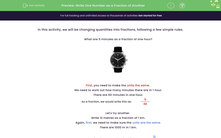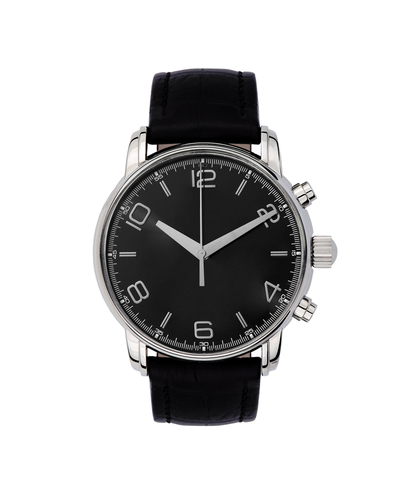# Write One Number as a Fraction of Another

In this worksheet, students will write one number as a fraction of another.Key stage:  KS 3

Curriculum topic:   Ratio, Proportion and Rates of Change

Curriculum subtopic:   Express One Quantity as a Fraction of Another

Difficulty level:#### Worksheet Overview

In this activity, we will be changing quantities into fractions, following a few simple rules.

What is 5 minutes as a fraction of one hour?First, we need to make the units the same.

We need to work out how many minutes there are in 1 hour.

There are 60 minutes in one hour.

As a fraction, we would write this as: 5/60

Let's try another.

Write 12 metres as a fraction of 1 km.

Again, first, we need to make sure the units are the same.

There are 1,000 m in 1 km.

As a fraction, we would write this as:  12/1,000

How do you feel about all that? Confident?!Let's try some questions.

### What is EdPlace?

We're your National Curriculum aligned online education content provider helping each child succeed in English, maths and science from year 1 to GCSE. With an EdPlace account you’ll be able to track and measure progress, helping each child achieve their best. We build confidence and attainment by personalising each child’s learning at a level that suits them.

Get started